# 智能音波测漏系统在长输天然气管道上的实践 Practice of Intelligent Sound Wave Leak Detection System in Long Distance Natural Gas Pipeline

DOI: 10.12677/JSTA.2020.83011, PDF, HTML, XML, 下载: 143  浏览: 515

Abstract: Pipeline leakage monitoring is of great significance to ensure the safe operation of natural gas long-distanced pipeline. This paper introduces the mechanism, composition and function of the intelligent sound wave leak detection system, and introduces the application of the system in Zhuhai Zhongshan Natural Gas Pipeline Project in detail. The results show that the intelligent acoustic wave leak detection system is accurate and effective in the long-distance natural gas pipeline testing, with high system robustness, quickly response, accurate positioning, and has practical significance.

1. 引言

2. 音波检漏系统介绍

2.1. 管道泄漏声波产生机理

2.2. Dolphin智能音波泄漏监测系统

Dolphin智能音波泄漏监测系统主要由：安装在管道站场和阀室的分站和安装在调度中心的主站组成。分站包括次声波传感器、现场数据采集处理器、系统控制主机(运行系统监控软件)等。经过多年的积累建立了强大的比对数据库作为诸多不同现场信号参照系，同时产品更适应TCP/IP协议下的新一代网络和技术。

3. 信号处理

3.1. 小波变换用于信号去噪

${\Psi }_{j,k}\left(t\right)={2}^{-\frac{j}{2}}\psi \left({2}^{-j}t-k\right),j,k\in Z$ (1)

$WT\left(j,k\right)={\int }_{-\infty }^{+\infty }x\left(t\right){\Psi }_{j,k}\left(t\right)\text{d}t$ (2)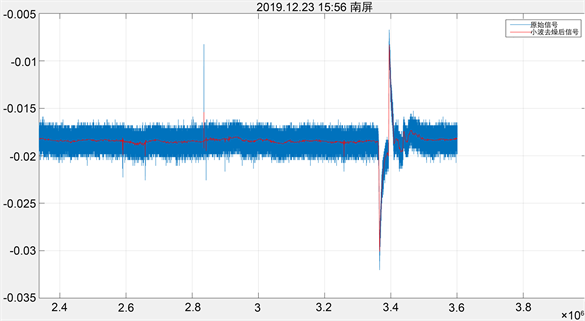Figure 1. 2019.12.23 15:56 Nanping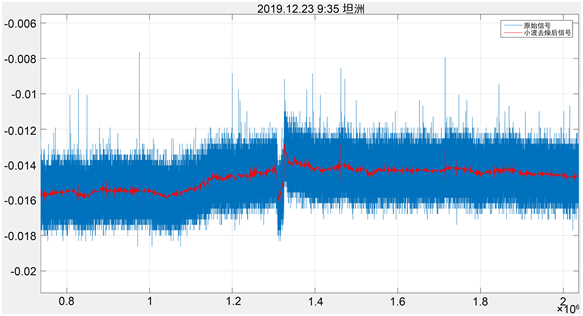Figure 2. 2019.12.23 9:35 Tanzhou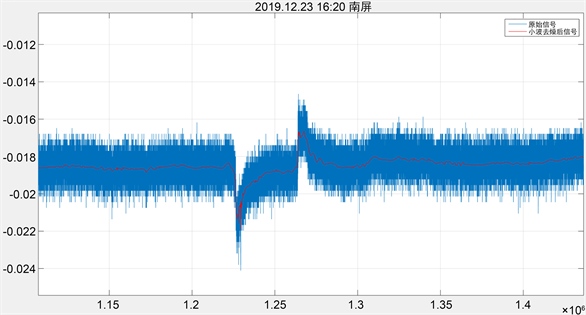Figure 3. 2019.12.23 16:20 Nanping

3.2. 微弱信号提取

$\stackrel{^}{w}=\underset{{‖w‖}^{2}=1}{\mathrm{arg}\mathrm{min}}{w}^{T}{X}^{T}Xw$ (3)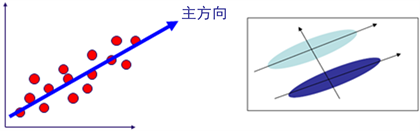Figure 4. PCA Illustration

$s\left(t\right)={\left[{s}_{1}\left(t\right),{s}_{2}\left(t\right),\cdots ,{s}_{M}\left(t\right)\right]}^{T}$ 为M个源信号， $x\left(t\right)={\left[{x}_{1}\left(t\right),{x}_{2}\left(t\right),\cdots ,{x}_{N}\left(t\right)\right]}^{T}$ 为N个观测信号。假设将M个 $s\left(t\right)$ 信号进行混合，形成N个 $x\left(t\right)$ 信号，其线性关系为

$x\left(t\right)=As\left(t\right)$ (4)

ICA方法就是需要从 $x\left(t\right)$ 中分离出独立源信号 $x\left(t\right)$。设ICA方法还原出的信号为 $y\left(t\right)={\left[{y}_{1}\left(t\right),{y}_{2}\left(t\right),\cdots ,{y}_{N}\left(t\right)\right]}^{T}$，其过程可描述为

$y\left(t\right)=Wx\left(t\right)=Ws\left(t\right)$ (5)

4. 音波测漏系统放气实验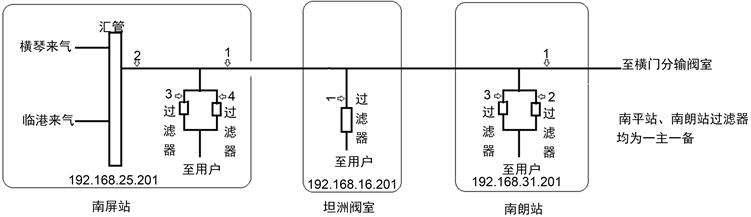Figure 5. Station (valve chamber) system construction drawing

Dolphin智能音波泄漏监测系统算法需要根据实际现场的数据信号进行调整识别来判断泄漏，然而不同的管道参数、运行环境不同，泄漏及其他操作产生的信号可能存在差异，因此需要模拟泄漏实验，为此提出选择该管道项目中的几条管道进行模拟放气泄漏实验，采集相应的信号波形，验证采集端对平稳工况的稳定性，对异常工况的敏感性。调整检测、定位算法参数，下面采用手轮转圈和孔板的两种模拟放气方式。Figure 6. Pipeline route

4.1. 手轮转圈模拟泄漏操作Table 1. Experimental record of hand wheel rotation deflation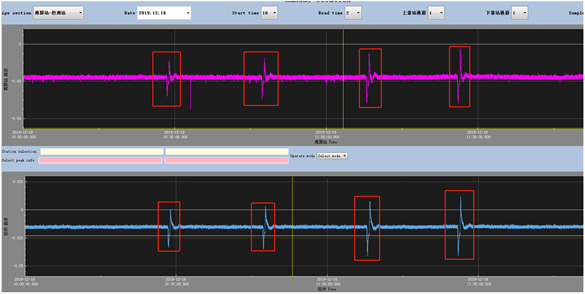Figure 7. 12.18 Data of Tanzhou outgassing experiment (Nanping Tanzhou)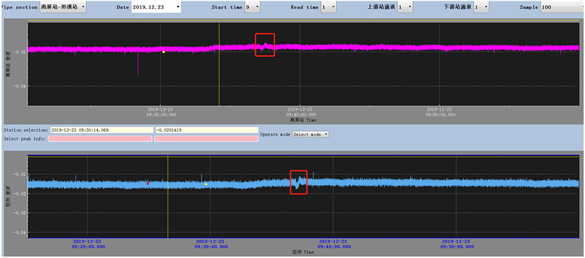Figure 8. 12.23 data of Nanlong outgassing experiment (Nanping Tanzhou)Figure 9. 12.23 data of Nanlong outgassing experiment (Tanzhou Nanlang)

4.2. 泄漏孔板模拟泄漏操作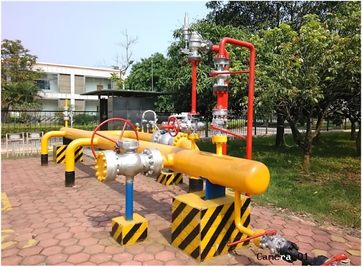Figure 10. The installation position of orifice plate device in Nanlang station is shown in the frame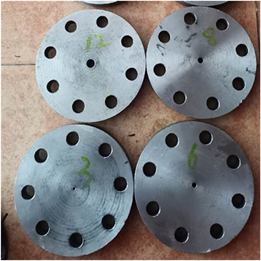Figure 11. Leakage orifice plate

Table 2. Experimental record of air release from leakage orifice plate

15:25:00放气60 s，孔径30 mm，实验数据如图12所示：南朗、坦洲泄漏信号均十分明显，有报警；

15:39:50放气90 s，孔径7 mm，实验数据如图13所示：南朗泄漏信号明显，尽管坦洲信号微弱，但是通过微弱信号提取技术，可以有效提取信号特征，提高泄漏报警效果。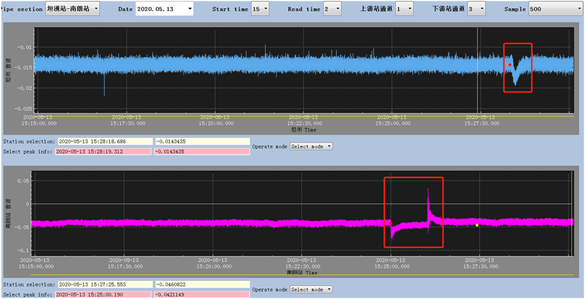Figure 12. 15:25:00 Nanlang outgassing test data and alarm record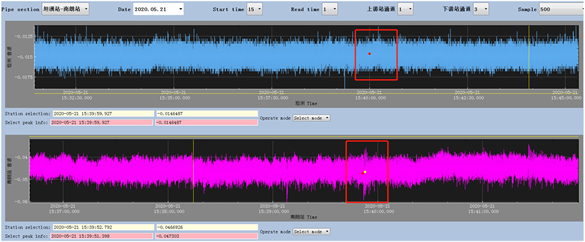Figure 13. 15: 39:50 Nanlang outgassing experiment data and alarm record (7 mm test)

4.3. 实验分析

1) 本次放气试验依据原同类产品测试方案进行测试，当时模拟放气是采用转圈方式，最小转圈数量为4圈，在五个测试点全部能检测到信号，从敏感性角度来说，与原同类产品结果类似；

2) 公司依据孔板测试方案进行了不同孔径的放气测试：50 mm、30 mm、12 mm、10 mm、8 mm、7 mm、6 mm。最小可检测孔径为7 mm，在南朗放气点(低压、远离主管) 7 mm泄漏孔径下测得的定位误差不超过50米，从敏感性角度来说，与竞争公司系统结果类似；

Dolphin智能音波泄漏监测系统可对微小泄漏信号检测、分析，通过泄漏实验校正，实现准确定位。

NOTES

*通讯作者。

  中国国家统计局. 2015年中国统计年鉴[M]. 北京: 中国国家统计局, 2015: 8-11.  靳世久, 孙家, 姜弘彦, 等. 强环境噪声下地下管道泄漏检测[J]. 天津大学学报: 自然科学与工程技术版, 1994(6): 782-787.  王海伟. 音波测漏系统在输气管道的应用[J]. 管道技术与设备, 2014(2): 22-24.  Yang, G., Liu, Y., Wang, Y., et al. (2015) EMD Interval Thresholding Denoising Based on Similarity Measure to Select Relevant Modes. Signal Processing, 109, 95-109. https://doi.org/10.1016/j.sigpro.2014.10.038  Wade, W.R. and Rachford, H.H. (1988) Detecting Leaks in Pipe Lines in Pipe Lines Using SCADA Information. Pipe Line Industry, 68, 64-66.  Wang, Z., Chang, J., Zhang, S., et al. (2015) An Improved Denoising Method in RDTS Based on Wavelet Transform Modulus Maxima. IEEE Sen-sors Journal, 15, 1061-1067. https://doi.org/10.1109/JSEN.2014.2360559  Li, S., Wen, Y., Li, P., et al. (2014) Modal Analysis of Leakage-Induced Acoustic Vibrations in Different Directions for Leak Detection and Location in Flu-id-Filled Pipelines. IEEE International Ultrasonics Symposium, Chicago, IL, 3-6 September 2014, 1412-1415. https://doi.org/10.1109/ULTSYM.2014.0349  郭静波, 蔡雄, 胡铁华, 张志文. 油气管道中智能机器人跟踪定位关键技术综述[J]. 仪器仪表学报, 2015(36): 481-498.  魏明生, 童敏明, 訾斌, 唐守锋. 基于粒子群-拟牛顿混合算法的管道机器人定位[J]. 仪器仪表学报, 2012(33): 2594-2600.  郭晨城, 文玉梅, 李平, 等. 采用EMD的管道泄漏声信号增强[J]. 仪器仪表学报, 2015, 36(6): 1397-1405.  Demirci, S., Yigit, E., Eskidemir, I.H., et al. (2012) Ground Penetrating Radar Imaging of Water Leaks from Buried Pipes Based on Back-Projection Method. Ndt & E International, 47, 35-42. https://doi.org/10.1016/j.ndteint.2011.12.008  孙洁娣, 肖启阳, 温江涛, 等. 局域均值分解分析的管道泄漏孔径识别及定位[J]. 仪器仪表学报, 2014, 35(12): 2835-2842.  曹欣雨. 自我健康监护仪软件系统设计及实现[D]: [硕士学位论文]. 长春: 长春理工大学, 2019.